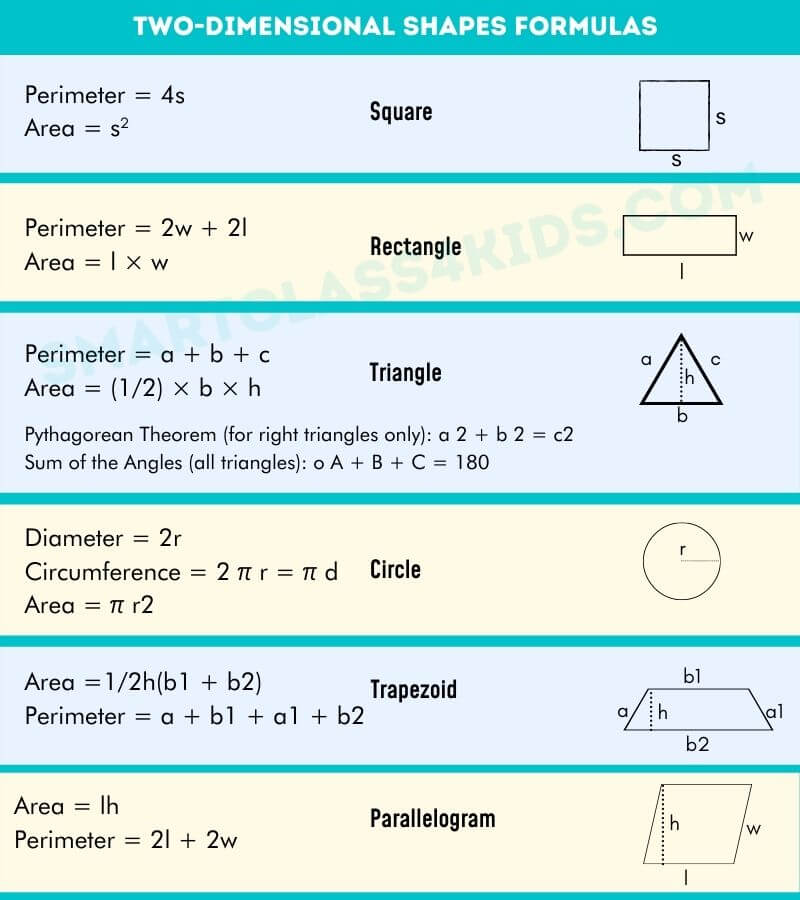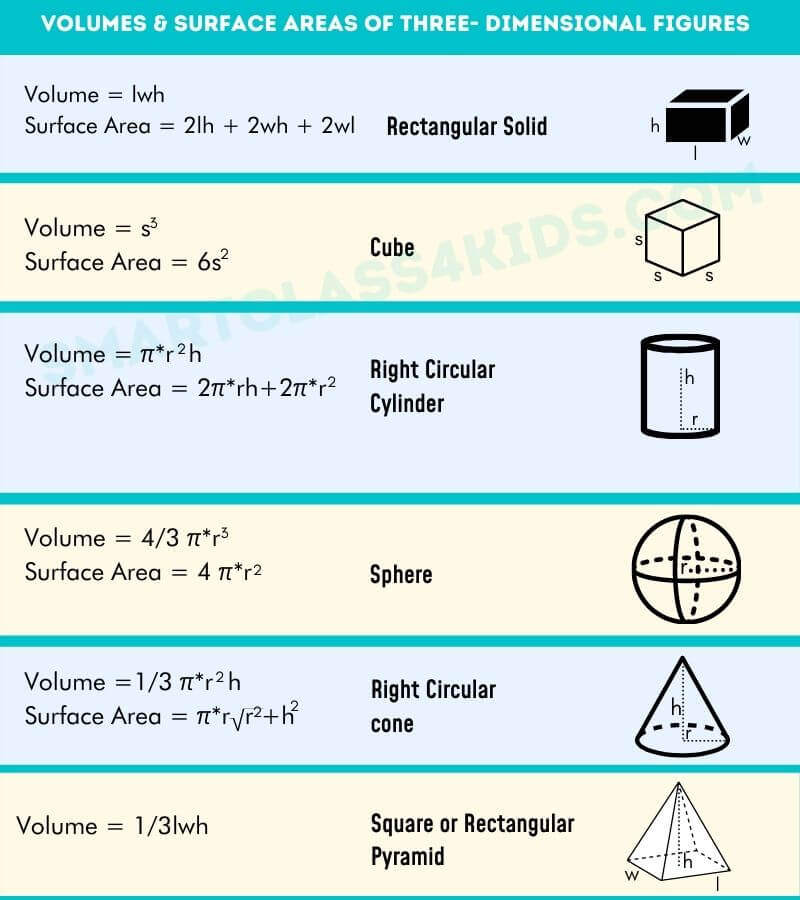# Basic Geometry Formulas

“These are some of the most common geometry formulas to figure out the perimeter, area, volume, surface areas, and circumference for the different shapes such as square, rectangle, triangle, parallelogram, circle, trapezoid etc.”
• Square
Perimeter = 4s
Area: A = s2
• Rectangle
Perimeter= 2w + 2l
Area= l × w
• Triangles
Perimeter = a + b + c
Area = (1/2) × b × h
• Circle
Diameter = 2r
Circumference = 2 π r = π d
Area = π r2
• Trapezoid
Area =1/2h(b1 + b2)
Perimeter = a + b1 + c + b2
• Isosceles Trapezoid
Perimeter = 2w + b1 + b2
Area = 1/2h(b1 + b2)
• Parallelogram
Area = lh
Perimeter = 2l + 2w

## Basic Geometry Formulas### TRY IT OUT

1. Two sides of a triangle are 7 and 13 centimeters. The perimeter is 27 centimeters. Find the third side.
2. If a square has an area of 49 ft2, what is the length of one of its sides? The perimeter?
3. If a rectangle has a width of 4, how long must its length be so that the area is 36?
4. If one angle of a right triangle is 70 degree, what are the other 2 angles.
5. What is the diameter of a circle with an area of 16 π ?
6. What is the circumference of the circle in problem 5? (suggest π = 3.14)

1- 7
2- side=7, perimeter=28
3- 9
4- 20
5- 8
6- 25.13

Scroll to Top